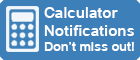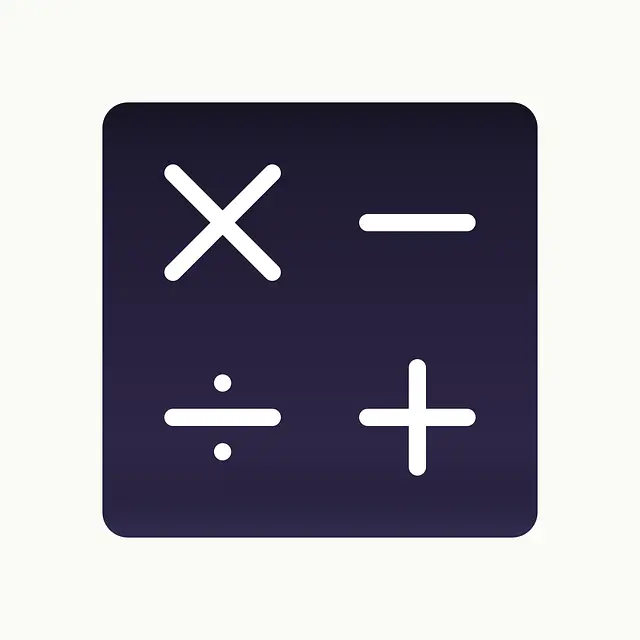Calculator Library
Free Online CalculatorsHome » 87 Calculators » Blog » What Is A Four Function Calculator?

Huge Domain Name Sale | Free Domains
Domain names on sale at crazy prices. Register a new domain for FREE when you buy another service.

## WHAT IS A FOUR FUNCTION CALCULATOR?Do you remember the good old days when we only had to use a four-function calculator for math class? These days, it seems like everyone is using their phones for everything, including math — but what is a four-function calculator and what can you do with it? In this article, we'll discuss the history of the four-function calculator and what each of its functions is. We'll also show you how to use a four-function calculator so that you can become a master of this nostalgic tool!

## What Is A Calculator?

A calculator is a device that's used to perform mathematical calculations. The first calculators were created in the early 1800s and they could only be used by mathematicians and scientists. However, as technology progressed, calculators became more and more available to the general public. The first four-function calculator was created in the 1970s and it quickly became the most popular type of calculator.

### Types Of Calculators

There are many different types of calculators available on the market today.

- Scientific Calculator: A scientific calculator is a type of calculator designed for more complex mathematical calculations.

- Graphing Calculator: A graphing calculator is a type of calculator that can graph equations.

- Financial Calculator: A financial calculator is a type of calculator that's designed to help with financial calculations.

## What Is A Four Function Calculator?

A four-function calculator is a type of calculator that can perform the four basic arithmetic operations: addition, subtraction, multiplication and division. Four function calculators usually have a small display screen and a few buttons that are used to enter numbers and symbols. Some four-function calculators also have memory functions so that you can store numbers for later use.

## What Are The Functions Of A Four Function Calculator?A four-function calculator has four main functions:

- Subtraction: This function allows you to subtract one number from another.

- Multiplication: This function allows you to multiply two numbers together.

- Division: This function allows you to divide one number by another.

These are the most basic mathematical operations and they can be performed with a four-function calculator. In addition to these four functions, a four-function calculator also has the square root and percentage functions.

## How Do You Use A Four-Function Calculator?

Using a four-function calculator is easy! To perform addition, subtraction, multiplication or division, simply enter the numbers that you want to calculate and press the corresponding button. For example, if you wanted to add two plus three, you would enter “two” followed by the addition button (+) and then “three”. The answer would then appear on the display screen.

To use the square root function, enter the number that you want to find the square root of and press the square root button. For example, if you wanted to find the square root of nine, you would “nine” and press the square root button. The answer would then appear on the display screen.

To use the percentage function, enter the number that you want to find the percentage of and press the percentage button. For example, if you wanted to find what 20% of 50 is, you would enter “20” followed by the percentage button (%) and then “50”. The answer would then appear on the display screen.

## When Can You Use A Four-Function Calculator?

A four-function calculator can be used for a variety of tasks, including:

- Basic arithmetic: You can use a four-function calculator for basic addition, subtraction, multiplication and division.

- Square roots: You can use a four-function calculator to find the square root of a number.

- Percentages: You can use a four-function calculator to calculate percentages.

## Limitations Of A Four-Function Calculator

While a four-function calculator is a great tool, it does have its limitations. For example, a four-function calculator cannot be used for more complex mathematical operations such as calculus or trigonometry. Additionally, four-function calculators usually do not have as many features as scientific or graphing calculators.

Despite its limitations, a four-function calculator is a handy tool to have around and can be used for a variety of tasks.

## Four Function Calculator Vs. Scientific Calculator

The main difference between a four-function calculator and a scientific calculator is that a scientific calculator can perform more complex mathematical operations such as calculus and trigonometry. Additionally, scientific calculators usually have more features than four-function calculators.

Both types of calculators are useful tools but it's important to choose the right type of calculator for the task at hand. If you need to perform complex mathematical operations, a scientific calculator is the better option. However, if you only need to perform basic arithmetic or square roots, a four-function calculator will suffice.

## Four Function Calculator Vs. Graphing Calculator

The main difference between a four-function calculator and a graphing calculator is that a graphing calculator can graph equations, while a four-function calculator cannot. A graphing calculator also usually has more features than a four-function calculator, such as the ability to solve equations and calculate derivatives.

A graphing calculator is a more versatile tool than a four-function calculator but it's not necessary for everyone. If you only need to perform basic arithmetic or square roots, a four-function calculator will suffice. However, if you need to graph equations or solve complex mathematical problems, a graphing calculator is the better option.

## Choosing The Right Calculator

When choosing a calculator, it's important to consider what you'll use it for. If you only need to perform basic arithmetic, a four-function calculator will suffice. However, if you need to perform more complex mathematical operations or graph equations, you'll need a scientific or graphing calculator.

## Conclusion

A four-function calculator is a simple yet powerful tool that can be used for a variety of mathematical operations. Whether you're a student who needs to do math homework or a professional who needs to calculate numbers for work, a four-function calculator is a great tool to have on hand. Now that you know how to use a four-function calculator, go ahead and try it out for yourself! Who knows, you might just become a master of this nostalgic tool!

 Previous Post« What Is A CAS Calculator? Next PostWhat Is A Non-Programmable Calculator? »